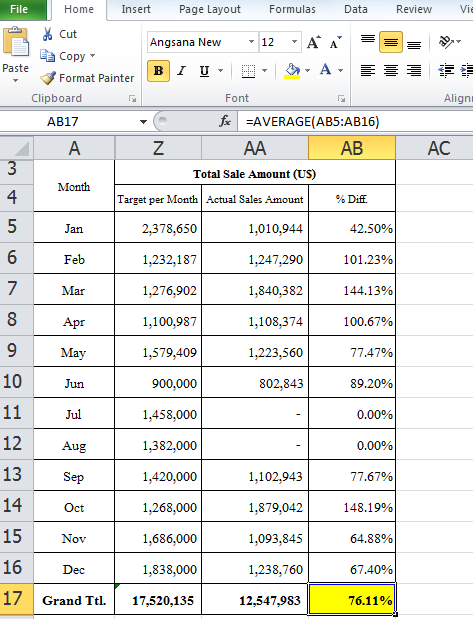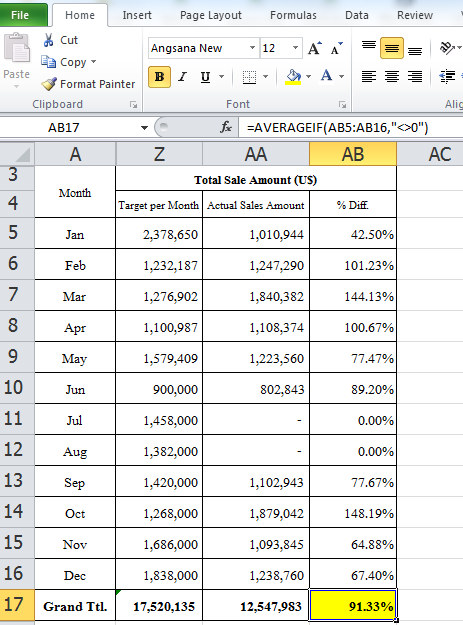371

# Compare Percentage Result Between Excel Formula [Average] And [Averageif Exclude “0”]

Do you know the result of percentage not the same if there is “0” figure in the Range of Average Formula?

Therefore, how do you solve this problem?

To obviously visible what the difference of using Average Formula between the range of figure “Including 0” and “Exclude 0”.

1] Let us have a look the first sample. We have the table of the whole year of sales amount. However, there are the two months, which are with the “0” sales amount, July and August.We use Excel formula “=AVERAGE(AB5:AB16)” to calculate the percentage average from January-December. The outcome is 76.11%. That would be true?

2] The second sample, we use all the same data as the number one. By the way, we have changed the formula to use “=AVERAGEIF(AB5:AB16,”<>0″)” instead. Here we gain 91.33%It does not matter what is our calculation criteria. Here, my article is just only to show you how the two formula work in different way. You can pick any of them and use it as you want.